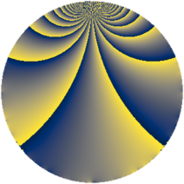# Properties

 Label 770.2.oLevel $770$ Weight $2$ Character orbit 770.o Rep. character $\chi_{770}(439,\cdot)$ Character field $\Q(\zeta_{6})$ Dimension $96$ Newform subspaces $2$ Sturm bound $288$ Trace bound $2$

# Related objects

## Defining parameters

 Level: $$N$$ $$=$$ $$770 = 2 \cdot 5 \cdot 7 \cdot 11$$ Weight: $$k$$ $$=$$ $$2$$ Character orbit: $$[\chi]$$ $$=$$ 770.o (of order $$6$$ and degree $$2$$) Character conductor: $$\operatorname{cond}(\chi)$$ $$=$$ $$385$$ Character field: $$\Q(\zeta_{6})$$ Newform subspaces: $$2$$ Sturm bound: $$288$$ Trace bound: $$2$$ Distinguishing $$T_p$$: $$17$$

## Dimensions

The following table gives the dimensions of various subspaces of $$M_{2}(770, [\chi])$$.

Total New Old
Modular forms 304 96 208
Cusp forms 272 96 176
Eisenstein series 32 0 32

## Trace form

 $$96q - 48q^{4} - 12q^{5} - 56q^{9} + O(q^{10})$$ $$96q - 48q^{4} - 12q^{5} - 56q^{9} + 2q^{11} + 4q^{14} + 8q^{15} - 48q^{16} - 12q^{26} + 24q^{31} + 112q^{36} + 2q^{44} + 12q^{45} - 72q^{49} - 8q^{56} - 4q^{60} + 96q^{64} - 48q^{66} - 4q^{70} - 80q^{71} + 180q^{75} + 12q^{80} - 144q^{81} - 24q^{86} + 120q^{89} - 112q^{91} - 116q^{99} + O(q^{100})$$

## Decomposition of $$S_{2}^{\mathrm{new}}(770, [\chi])$$ into newform subspaces

Label Dim. $$A$$ Field CM Traces $q$-expansion
$$a_2$$ $$a_3$$ $$a_5$$ $$a_7$$
770.2.o.a $$48$$ $$6.148$$ None $$-24$$ $$0$$ $$-6$$ $$-4$$
770.2.o.b $$48$$ $$6.148$$ None $$24$$ $$0$$ $$-6$$ $$4$$

## Decomposition of $$S_{2}^{\mathrm{old}}(770, [\chi])$$ into lower level spaces

$$S_{2}^{\mathrm{old}}(770, [\chi]) \cong$$ $$S_{2}^{\mathrm{new}}(385, [\chi])$$$$^{\oplus 2}$$